Navier-Stokes equations

(Redirected from Navier–Stokes equations)

The fundamental equations of motion of a viscous liquid; they are mathematical expressions of the conservation laws of momentum and mass. For a non-stationary flow of a compressible liquid, the Navier–Stokes equations in a Cartesian coordinate system may be written as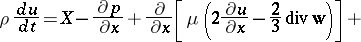(1)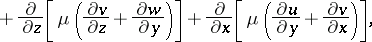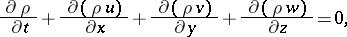where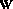is the velocity vector, with projections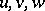on the coordinate axes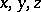, respectively;is the pressure,the density,the viscosity coefficient;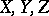are the projections of the mass force vectoron the coordinate axes; andis the substantive derivative. The derivation of equations (1) is based on Newton's generalized law of friction, according to which the stress in a moving fluid or gas is proportional to the strain rates. For the study of compressible flows one must add to (1) the equation of state, which relates the pressure, the density and the temperature, and the energy equation.

Equations (1), which are the basis of hydrodynamics, were first derived by L. Navier  and S.D. Poisson , on the basis of considerations involving the action of intermolecular forces. B. Saint-Venant  and G.G. Stokes  derived the equations under the sole assumption that the normal and tangential stresses are linear functions of the strain rates.

For a flow of an incompressible isothermic liquid, equations (1) can be put in the following vector form: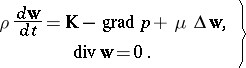(2)

As a rule, when analyzing the Navier–Stokes equations one transforms them to dimension-less form, scaling all quantities figuring therein by dividing by appropriate characteristic units. Thus, for stationary flows of an incompressible liquid, with no mass forces, the Navier–Stokes equations contain one decisive dimension-less parameter, known as the Reynolds number: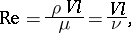where,are the characteristic velocity and linear dimension, andis the kinematic viscosity.

To study two-dimensional incompressible flows one frequently uses the Helmholtz form of the Navier–Stokes equations: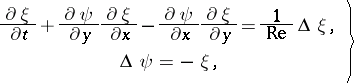(3)

whereis the stream function andthe rotation, which are related to the velocity projectionsandas follows:The fundamental boundary value problems for the stationary Navier–Stokes equations are those associated with the investigation of flows in closed cavities, channels, flows with free surfaces, flows around bodies, jet flows, and wakes behind bodies. In all these problems the equations are integrated over (finite or infinite) domains with boundary conditions determined by physical considerations (adhesion or slipping along the surfaces of bodies, blowing-in or suction through permeable surfaces, conditions governing the external flow far from the body in the flow, conditions on the free boundary, etc.). For non-stationary problems one needs, besides the boundary conditions, suitable initial conditions.

As yet (1989) there exists no rigorous mathematical analysis of the solvability of the boundary value problems of hydro- and aeromechanics for the Navier–Stokes equations. A few results are available in the mathematical theory of the dynamics of viscous incompressible liquids (see Hydrodynamics, mathematical problems in).

The efforts of investigators were originally directed towards the determination of exact solutions. For example, in the incompressible case there exist exact solutions for the following stationary flows: in a plane channel with given constant pressure differential (Poiseuille flow); between two parallel plane walls, one of which is at rest, while the other is moving in its own plane at constant velocity (Couette flow); in a rectilinear tube of circular cross-section with constant pressure drop (Hagen–Poiseuille flow). A few self-similar solutions have also been found, including: plane-parallel (and axially-symmetric) flow near a critical point (Howart flow); and flows in contracting and expanding channels (Hamel flow).

Approximate solutions of the Navier–Stokes equations are based on simplifying assumptions. Worthy of mention here are solutions for very small Reynolds numbers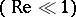, corresponding to creeping motions, the best-known of which is a Stokes flow around a sphere. The limiting case of very large Reynolds numbers leads to the theory of the hydrodynamic boundary layer. The boundary-layer equations have made it possible to solve a broad range of problems of practical importance, using well-developed approximate and numerical methods.

Certain classes of problems in the dynamics of viscous liquids and gases can be solved with the aid of fairly efficient algorithms, based on the use of difference schemes. For example, this is the case for the calculation of laminar flows of viscous incompressible liquids in regions of simple shape (or around bodies of simple shape). The most widely used difference methods in this context are those intended for the equations in Helmholtz form (3), though this system involves certain difficulties stemming from the determination of boundary conditions for. The first results in solving the stationary version of system (3) were obtained using simple explicit five-point schemes and iterative methods (see ). The solution of stationary problems in the dynamics of viscous incompressible liquids are largely based on the adjustment method and on the application of explicit and implicit schemes for the system (3). Of the explicit schemes, one uses schemes with two levels in time, with symmetric approximation of the first derivatives by central differences and the solution of the second equation in (3) on each time level by Seidel's method; also used is a three-level scheme in which the convective terms are approximated by a "cross" scheme and the diffusion terms by the Dufort–Frankel scheme. These schemes are also adequate to yield certain results in the solution of stationary problems for two-dimensional laminar flows in contracting and expanding channels, in a rectilinear cut with moving cover, and also in the non-stationary problem of flow in a channel around a flat plate perpendicular to the direction of the flow.

Implicit schemes are based, as a rule, on the method of fractional steps (see ). The general structure of these schemes for equations (3) may be presented, for example, as follows: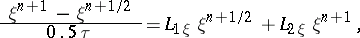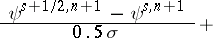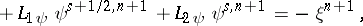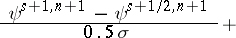where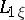,,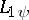,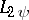are one-dimensional difference operators: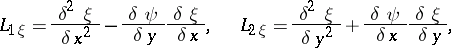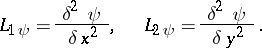In these formulas,is the time step;the iteration parameter;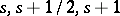are the iteration indices in the iterative solution of the Poisson equation in (3) on the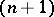-st time level; and,,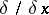,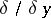are the difference operators approximating the corresponding second- and first-order derivatives. The first equation of (3) is used to find the values of, the second — to find the values ofon the next time level. The approximation of the second derivatives is usually symmetric, while that of the first derivatives in the equation foris done either with symmetric differences or with one-sided differences against the flow, with the sign of the velocity taken into consideration. A highly-recommended method is the scheme developed in , together with monotone approximation (see ). For the first equation of (3), this scheme is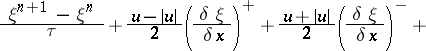(4)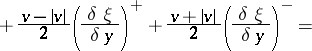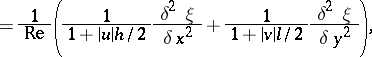whereandare the grid steps in the- and-directions, andThe difference equations (4) are usually transformed to three-diagonal form and solved, together with relations approximating the boundary conditions, by the double-sweep method. When solving stationary problems by the adjustment method one can either successively solve equations (4) (without internal iterations for the determination of) or simultaneously solve the equations of (4), determiningandjointly, by a vector double-sweep. The difficulty in the formulation of boundary conditions for equations (3) is that, in the case of the Navier–Stokes equations, the ordinary boundary conditions of adhesion at solid walls yield conditions only for. A formal requirement in the numerical solution of the equation foris the formulation of boundary conditions for the rotation. These conditions can be obtained on each time level, either approximately, on the boundary of the region, or by integrating the equation foronly in a region within the basic domain of integration .

The equations in the form (2) are less commonly used to study two-dimensional flows; usually, the continuity equation is regularized in some way. Variational-grid methods, in particular the method of finite elements, have found application in solving the equations of the dynamics of viscous fluids in the forms (2) and (3).

Difference methods have been applied to the investigation of a variety of flow problems for viscous incompressible liquids. Among these are flow around an elliptic or circular cylinder (including a rotating cylinder), around a plate of finite thickness (including a plate at an angle of attack), a cylindrical butt-end, a drop, a plane step, etc. Flows have also been studied in a cavity, in plane and cylindrical channels, in a channel with obstacles on the walls, as well as flows with a free surface, and flows of natural, induced and mixed convection.

Calculations for viscous compressible gases, based on the application of difference methods to the full Navier–Stokes equations, involve certain additional difficulties in comparison with calculations for viscous incompressible liquids. The reason is that in the flow of a compressible gas one has not only boundary-layer regions, but also other regions characterized by high gradients of the unknown functions corresponding to shock waves and rarefraction waves in the non-viscous gas flows. The complexity of the Navier–Stokes equations themselves for a viscous compressible gas imposes considerable demands on the speed and memory of the computer. In this case the application of explicit schemes yields simpler algorithms. One explicit scheme recommended for the calculation of stationary flows by the adjustment method is the scheme that, applied as an illustration to the simple equation with constant coefficients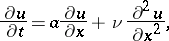(5)

may be written asThis scheme approximates (5) for a smooth steady-state solution, up to the order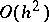, and is stable for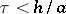. The stability condition is independent of. Applied to the Navier–Stokes equations, this means that the stability condition is independent of the Reynolds number. Nevertheless, the restriction imposed on the time iteration step in explicit schemes is substantial. Implicit schemes are usually free of this restriction and they are absolutely stable for such linear equations with constant coefficients. Implicit schemes for calculating two-dimensional flows of a viscous gas are used in combination with the method of fractional steps. Various difference schemes of the variable-directions method have recently (1982) been constructed: with complete and incomplete approximation on intermediate levels, divergent and non-divergent (see ), and schemes with an improved order of accuracy (higher than the second order) relative to the space step of the grid (see ). Numerical simulation of flows of a viscous gas based on the Navier–Stokes equations involves the calculation of flows of a complex structure and the use of sufficiently fine grids. This is impossible, because of limitations on the computer memory, without the use of the method of mutually overlapping regions (see ). Difference methods for solving the Navier–Stokes equations have been used to investigate a large number of problems in the dynamics of a viscous gas. Among these are: supersonic flow around blunt bodies (spheres, butt-ends of a circular cylinder), around compound bodies (sphere-cylinder, sphere-cone), and around a wedge or a leading edge of a flat plate. Flows have also been considered in wakes behind bodies of finite dimensions, in nozzles and air intakes, and in cavities with external sub- and supersonic current. Other topics that have been studied are the interaction of a boundary layer with a shock wave, the structure of a shock wave in a plasma, etc. The flows mentioned above are usually assumed to be laminar.